# Determination of Heat Capacity Using Phase Changes

Using differential scanning calorimetry (DSC) it is possible to measure the specific heat capacity using different methods. The direct method and the sapphire method are commonly used and it should be noted that the sapphire method provides reliable results especially for temperatures above 1600°C. Applications of the sapphire method are discussed in this paper.

## Determination of Cp Changes

The examples that follow show various application possibilities of the sapphire method for the determination of Cp at high temperatures. The measurements were done with the use of a Mettler Toledo TGA/DSC 1 equipped with an HSS2 sensor. For all the experiments a platinum crucible with lid was used. The curves are blank corrected.

## Change in Cp at the Glass Transition

There is a marked change in the specific heat capacity during glass transition. With the help of the sapphire method, it is possible to measure this change with a glass sample as shown in Figure 1.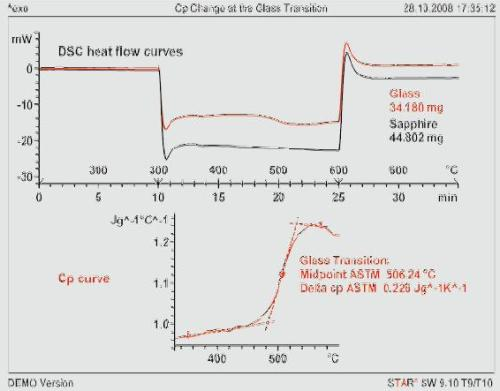Figure 1. DSC curves of a glass sample and sapphire to determine the glass transition and the change in Cp of the glass. Heating rate 10 K/min; crucible 30-μL Pt.

It is possible to read the characteristic glass transition temperature from the DSC curve, whereas the overall course of the Cp and the magnitude of the change at the glass transition is possible only using the Cp curve.

## Change in Cp in Second Order Phase Transitions

Second order phase transitions show a distinctive change in the specific heat capacity without any involvement of latent heat or additional energy. The change is noted as an apparent endothermic effect on the DSC heating curve of a suitable sample. The dependence of the specific heat capacity is measured using the sapphire method. The example shown in Figure 2 displays the Cp function of cobalt at the Curie point at 1120 °C (1393 K). The Cp curve shows a typical l-shape. The measurement values lie in the range of literature values for cobalt and the standard deviation is about ± 10%.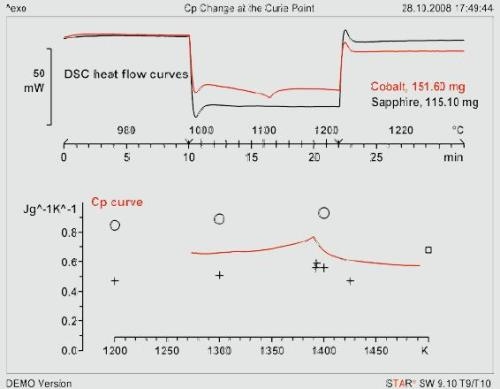Figure 2. Upper diagram: DSC curves of cobalt and sapphire using the sapphire method. Lower diagram: the Cp curve, literature values values: o, + , □ . Cobalt measured as five disks. Heating rate 20 K/min; crucible 30-µL Pt with lid.

## Change in Cp in Decomposition Reactions

While measuring Cp, it is assumed that the sample does not change, but since this does not happen in a decomposition reaction the sapphire method would measure an apparent specific heat capacity. It is important to consider the change in mass to measure Cp of the start and end product.

By using TGA and DSC techniques in the TGA/DSC 1 mass and enthalpy changes are simultaneously measured using the same test specimen.

## Example to Study Cp in Decomposition Reactions

The thermal decomposition of calcium carbonate to calcium oxide is studied as an example. The heat flow determined during the decomposition of calcium carbonate includes a latent heat component that is measured by the reaction enthalpy. The TGA and DSC curves are shown in Figure 3.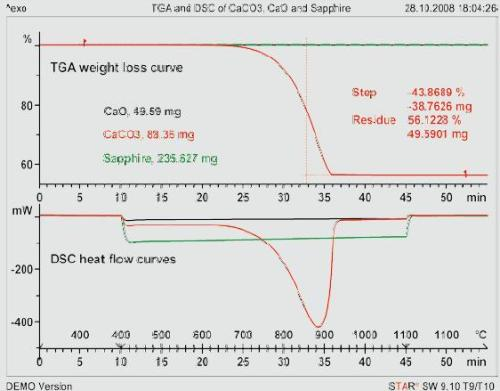Figure 3. TGA and DSC curves of sapphire, CaCO3 and CaO measured according to the sapphire method. Heating rate 20 K/min; crucible: 150-μL Pt with lid.

Pure calcium carbonate in a nitrogen atmosphere decomposes to calcium oxide that is left behind as a residue. There is a mass loss of 43.97%. The specific heat capacity of CaCO3 measured before decomposition that starts at about 600 °C agrees well with the literature value as shown in Figure 4.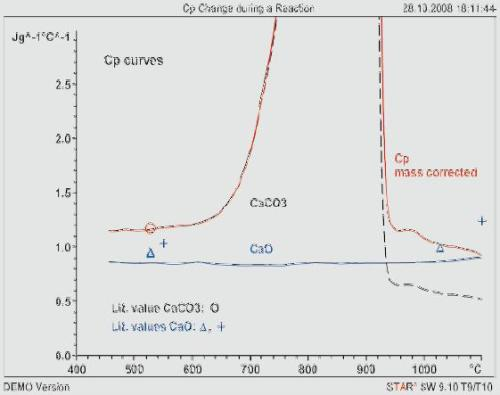Figure 4. The Cp curves of CaCO3 and CaO calculated from the curves in Figure 3 using the sapphire method. Black dashed line: Cp without mass correction; red line with correction. Literature data: + , Δ , o [5, 6].

Observations from the example are listed below:

• There is an increase in Cp because of the overlapping decomposition enthalpy. The curve corresponds to the apparent specific heat capacity.
• After the reaction completion, the Cp value measured is too low if normalization is performed as usual with respect to the original starting mass (dashed curve).
• The STARe software enables the Cp calculation to be performed with respect to the changing mass. The result of this calculation is shown in Figure 4 as the red curve that is mass corrected. It shows that correct values are obtained for the calcium oxide.
• Another heating run of the same test specimen as shown in Figure 3 produced the Cp curve of CaO for the complete temperature range as shown by the blue curve in Figure 4.
• There is no mass change hence calculation is made with constant starting mass. In the example shown, this corresponds to the residue from the first heating run.
• At 1100 °C, the Cp values of both measurements agree well.

## Determination of the Process Enthalpy from Cp Measurements

The process enthalpy is the sum of the heat needed to heat the material called the sensible heat and the latent heat or the reaction enthalpy. It is possible to calculate both these values from the Cp curve but the process enthalpy is studied with respect to initial mass.

The enthalpy and its change as a function of temperature are determined by integrating the Cp curve.

## Example for Determining Process Enthalpy

The calcination of CaCO3 is again considered as an example. In Figure 5, the Cp curve calculated with reference to the starting material is shown.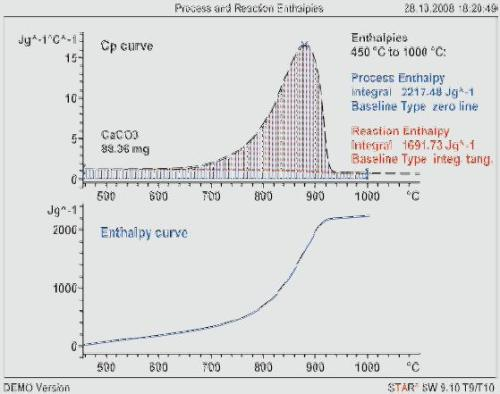Figure 5. The Cp curve of CaCO3 (dashed, not mass corrected). The blue curve is the enthalpy as a function of temperature.

Observation from the example is listed below:

• Integration with reference to temperature gives a process enthalpy of approx. 2217 J/g for the calcinations between 450 and 1000°C.
• The enthalpy is calculated as a function of the temperature shown by the blue curve by partial integration of the Cp curve, and the starting value is set to zero.
• By integration of the Cp curve above the red baseline, the reaction enthalpy is obtained
• This gives a value of approx. 1692 J/g. The baseline is constructed assuming that the Cp value of the sample changes proportional to the conversion.

## Summary and Conclusions

The change in Cp occurring during different thermal events are studied. The glass transition of an inorganic glass and the second order phase transition of cobalt are shown as examples. The sample mass normally remains constant during determination of Cp. If a chemical or physical change involving latent heat takes place, an apparent heat capacity is determined. In a decomposition reaction, there is a change in the mass of the sample. This mass change must be considered in order to determine correct Cp values of the starting and end products. The integration of the Cp curve with respect to temperature yields the enthalpy of a process. This was shown using the calcination of CaCO3 as an example.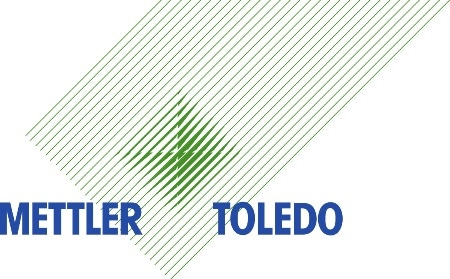This information has been sourced, reviewed and adapted from materials provided by Mettler Toledo - Thermal Analysis.

## Citations

• APA

Mettler Toledo - Thermal Analysis. (2020, January 07). Determination of Heat Capacity Using Phase Changes. AZoM. Retrieved on March 28, 2020 from https://www.azom.com/article.aspx?ArticleID=5743.

• MLA

Mettler Toledo - Thermal Analysis. "Determination of Heat Capacity Using Phase Changes". AZoM. 28 March 2020. <https://www.azom.com/article.aspx?ArticleID=5743>.

• Chicago

Mettler Toledo - Thermal Analysis. "Determination of Heat Capacity Using Phase Changes". AZoM. https://www.azom.com/article.aspx?ArticleID=5743. (accessed March 28, 2020).

• Harvard

Mettler Toledo - Thermal Analysis. 2020. Determination of Heat Capacity Using Phase Changes. AZoM, viewed 28 March 2020, https://www.azom.com/article.aspx?ArticleID=5743.

## Tell Us What You Think